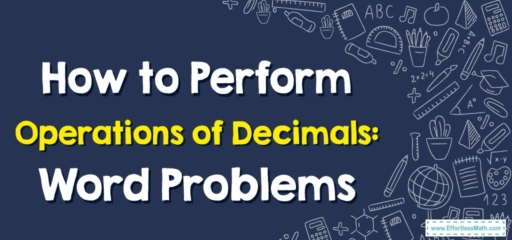# How to Perform Operations of Decimals: Word Problems

Greetings, budding mathematicians! Today, we're going to jump into an adventure with decimals. Working with decimals is a fundamental math skill that you'll use in many aspects of life, from managing money to measuring distances.## Introduction to Decimal Operations

First things first, let’s talk about decimals. A decimal is a way of representing a number that’s less than one, or a number that’s a whole and a fraction together. When you work with decimals, the same rules apply as when you’re working with whole numbers, but the decimal point plays a crucial role.

There are four major operations that you can perform on decimals:

2. Subtraction
3. Multiplication
4. Division

Now, let’s tackle some word problems involving decimal operations.

## Step-By-Step Guide to Solving Word Problems with Decimal Operations

### Step 1: Understand the Problem

First and foremost, carefully read through the problem. Identify what you know and what you need to find out.

### Step 2: Plan the Solution

Next, decide which operation (addition, subtraction, multiplication, or division) will help you solve the problem. This will depend on what the problem is asking.

### Step 3: Carry Out the Operation

Perform the operation on the decimals. Remember to align the decimal points when you’re adding or subtracting, and apply the rules for multiplication and division of decimals correctly.

### Step 4: Check the Answer

Finally, check if your answer makes sense in the context of the problem.

### Example:

Consider this problem: Laura bought $$2.3$$ kilograms of apples and $$1.5$$ kilograms of grapes. How many kilograms of fruit did she buy in total?

Step 1: Understand that you know the weight of apples and grapes separately, and you need to find the total weight.

Step 2: Realize that to find the total, you need to add the weights together.

Step 3: Add the weights: $$2.3\ kg + 1.5\ kg = 3.8\ kg$$.

Step 4: Check that the answer makes sense. Laura bought $$2.3\ kg$$ of apples and $$1.5\ kg$$ of grapes, so it makes sense that she bought $$3.8\ kg$$ in total.

Keep practicing with different word problems, and you’ll get the hang of decimal operations in no time. Remember, the more you practice, the better you’ll get. Happy calculating!

### What people say about "How to Perform Operations of Decimals: Word Problems - Effortless Math: We Help Students Learn to LOVE Mathematics"?

No one replied yet.

X
30% OFF

Limited time only!

Save Over 30%

SAVE $5 It was$16.99 now it is \$11.99